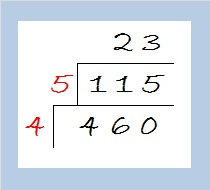460 Happy Birthday, Tim!

460 is the sum of consecutive prime numbers. Check the comments because one of my readers was able to find what those consecutive primes are.

Happy birthday to my son, Tim. I have two different cakes for you in this post. A cake puzzle and a simplified square root that uses the cake method that I’ve modified.This puzzle will be included in an excel file of puzzles 12 Factors 2015-04-20.

—————————————————————————————————

When we simplify square roots, we want to do as few divisions as possible. Since 60 can be evenly divided by perfect square 4, we know that 460 is also divisible by 4. Let’s use that fact to find its square root:The quotient, 115, may be too large for us to know if it has any square factors. Since it isn’t divisible by 4, 9, or 25, let’s make a second layer to our cake as we divide it by its largest prime factor, 5.Since the new quotient, 23, is a prime number, let’s revert back to the previous cake and take the square root of everything on the outside of the one layer cake: √460 = (√4)(√115) = 2√115.

• 460 is a composite number.
• Prime factorization: 460 = 2 x 2 x 5 x 23, which can be written 460 = (2^2) x 5 x 23
• The exponents in the prime factorization are 2, 1, and 1. Adding one to each and multiplying we get (2 + 1)(1 + 1)(1 + 1) = 3 x 2 x 2 = 12. Therefore 460 has exactly 12 factors.
• Factors of 460: 1, 2, 4, 5, 10, 20, 23, 46, 92, 115, 230, 460
• Factor pairs: 460 = 1 x 460, 2 x 230, 4 x 115, 5 x 92, 10 x 46, or 20 x 23
• Taking the factor pair with the largest square number factor, we get √460 = (√4)(√115) = 2√115 ≈ 21.4476—————————————————————————————————Here’s the order the factors were found:2 thoughts on “460 Happy Birthday, Tim!”

1.wbhs62

Happy birthday, Tim!

460 = 17+19+23+29+31+37+41+43+47+53+59+61

•ivasallay

Thank you.

Good job finding those 12 consecutive prime numbers that add up to 460, too!

This site uses Akismet to reduce spam. Learn how your comment data is processed.